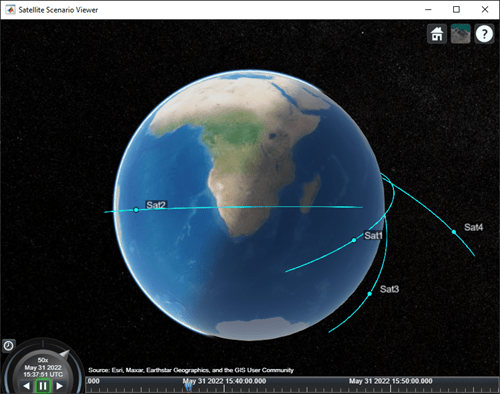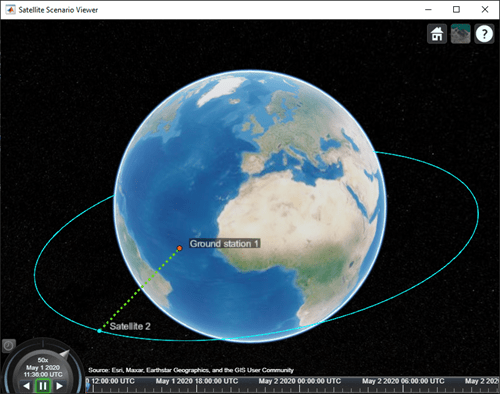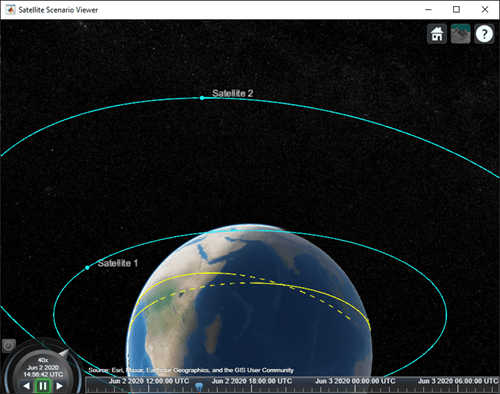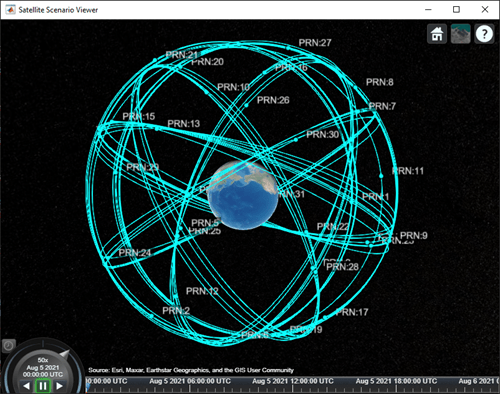# satellite

Since R2021a

## Syntax

``satellite(scenario,file)``
``satellite(scenario,RINEXdata)``
``satellite(scenario,semimajoraxis,eccentricity,inclination,RAAN,argofperiapsis,trueanomaly)``
``satellite(scenario,positiontable)``
``satellite(scenario,positiontable,velocitytable)``
``satellite(scenario,positiontimeseries)``
``satellite(scenario,positiontimeseries,velocitytimeseries)``
``satellite(___,Name,Value)``
``sat = satellite(___)``

## Description

example

````satellite(scenario,file)` adds `Satellite` objects from `file` to the satellite scenario specified by `scenario`. The yaw (z) axes of the satellites point toward nadir and the roll (x) axes of the satellites align with their respective inertial velocity vectors. ```
````satellite(scenario,RINEXdata)` adds `Satellite` objects from `RINEXdata` to the satellite scenario specified by `scenario`.```
````satellite(scenario,semimajoraxis,eccentricity,inclination,RAAN,argofperiapsis,trueanomaly)` adds a `Satellite` object from Keplerian elements defined in the Geocentric Celestial Reference Frame (GCRF) to the satellite scenario.```

example

````satellite(scenario,positiontable)` adds a `Satellite` object from position data specified in `positiontable` (`timetable` object) to the scenario. This function creates a `Satellite` with `OrbitPropagator="ephemeris"`.```

example

````satellite(scenario,positiontable,velocitytable)` adds a `Satellite` object from position data specified in `positiontable` (`timetable` object) and velocity data specified in `velocitytable` (`timetable` object) to the scenario. This function creates a `Satellite` with `OrbitPropagator="ephemeris"`.```
````satellite(scenario,positiontimeseries)` adds a `Satellite` object from position data specified in `positiontimeseries` to the `scenario`. This function creates a `Satellite` with `OrbitPropagator="ephemeris"`.```
````satellite(scenario,positiontimeseries,velocitytimeseries)` adds a `Satellite` object to the `scenario` from position (in meters) data specified in `positiontimeseries` (`timeseries` object) and velocity (in meters/second) data specified in `velocitytimeseries` (`timeseries` object). This function creates a `Satellite` with `OrbitPropagator="ephemeris"`.```
````satellite(___,Name,Value)` specifies options using one or more name-value arguments in addition to any input argument combination from previous syntaxes. ```
````sat = satellite(___)` returns a vector of handles to the added satellites. Specify any input argument combination from previous syntaxes. NoteWhen the `AutoSimulate` property of the `satelliteScenario` is `false`, you can modify the `satellite` only when the `SimulationStatus` is `NotStarted`. You can use the `restart` function to reset `SimulationStatus` to `NotStarted`, but doing so erases the simulation data. ```

## Examples

collapse all

Create a satellite scenario object.

`sc = satelliteScenario;`

Load the satellite ephemeris timetable in the Earth Centered Earth Fixed (ECEF) coordinate frame.

`load("timetableSatelliteTrajectory.mat","positionTT","velocityTT");`

Add four satellites to the scenario.

`sat = satellite(sc,positionTT,velocityTT,"CoordinateFrame","ecef");`

Visualize the trajectories of the satellites.

`play(sc);`Create a satellite scenario and add ground stations from latitudes and longitudes.

```startTime = datetime(2020,5,1,11,36,0); stopTime = startTime + days(1); sampleTime = 60; sc = satelliteScenario(startTime,stopTime,sampleTime); lat = 10; lon = -30; gs = groundStation(sc,lat,lon);```

```semiMajorAxis = 10000000; eccentricity = 0; inclination = 10; rightAscensionOfAscendingNode = 0; argumentOfPeriapsis = 0; trueAnomaly = 0; sat = satellite(sc,semiMajorAxis,eccentricity,inclination, ... rightAscensionOfAscendingNode,argumentOfPeriapsis,trueAnomaly);```

Add access analysis to the scenario and obtain the table of intervals of access between the satellite and the ground station.

```ac = access(sat,gs); intvls = accessIntervals(ac)```
```intvls=8×8 table Source Target IntervalNumber StartTime EndTime Duration StartOrbit EndOrbit _____________ __________________ ______________ ____________________ ____________________ ________ __________ ________ "Satellite 2" "Ground station 1" 1 01-May-2020 11:36:00 01-May-2020 12:04:00 1680 1 1 "Satellite 2" "Ground station 1" 2 01-May-2020 14:20:00 01-May-2020 15:11:00 3060 1 2 "Satellite 2" "Ground station 1" 3 01-May-2020 17:27:00 01-May-2020 18:18:00 3060 3 3 "Satellite 2" "Ground station 1" 4 01-May-2020 20:34:00 01-May-2020 21:25:00 3060 4 4 "Satellite 2" "Ground station 1" 5 01-May-2020 23:41:00 02-May-2020 00:32:00 3060 5 5 "Satellite 2" "Ground station 1" 6 02-May-2020 02:50:00 02-May-2020 03:39:00 2940 6 6 "Satellite 2" "Ground station 1" 7 02-May-2020 05:59:00 02-May-2020 06:47:00 2880 7 7 "Satellite 2" "Ground station 1" 8 02-May-2020 09:06:00 02-May-2020 09:56:00 3000 8 9 ```

Play the scenario to visualize the ground stations.

`play(sc)`Create a satellite scenario with a start time of 02-June-2020 8:23:00 AM UTC, and the stop time set to one day later. Set the simulation sample time to 60 seconds.

```startTime = datetime(2020,6,02,8,23,0); stopTime = startTime + days(1); sampleTime = 60; sc = satelliteScenario(startTime,stopTime,sampleTime);```

Add two satellites to the scenario using their Keplerian elements.

```semiMajorAxis = [10000000;15000000]; eccentricity = [0.01;0.02]; inclination = [0;10]; rightAscensionOfAscendingNode = [0;15]; argumentOfPeriapsis = [0;30]; trueAnomaly = [0;20]; sat = satellite(sc,semiMajorAxis,eccentricity,inclination, ... rightAscensionOfAscendingNode,argumentOfPeriapsis,trueAnomaly)```
```sat = 1x2 Satellite array with properties: Name ID ConicalSensors Gimbals Transmitters Receivers Accesses GroundTrack Orbit OrbitPropagator MarkerColor MarkerSize ShowLabel LabelFontColor LabelFontSize ```

View the satellites in orbit and the ground tracks over one hour.

```show(sat) groundTrack(sat,LeadTime=3600)```
```ans=1×2 object 1×2 GroundTrack array with properties: LeadTime TrailTime LineWidth LeadLineColor TrailLineColor VisibilityMode ```

Play the scenario and set the animation speed of the simulation to 40.

`play(sc,PlaybackSpeedMultiplier=40)`Set up the satellite scenario.

```startTime = datetime(2021,8,5); stopTime = startTime + days(1); sampleTime = 60; % seconds sc = satelliteScenario(startTime,stopTime,sampleTime);```

Add satellites to the scenario from a SEM almanac file.

`sat = satellite(sc,"gpsAlmanac.txt","OrbitPropagator","gps");`

Visualize the GPS constellation.

`v = satelliteScenarioViewer(sc);`Add a satellite to a satellite scenario from a Consultative Committee for Space Data Systems (CCSDS) Orbit Mean-Elements Message (OMM) file.

Create a satellite scenario. Use the epoch defined in the OMM file as the start time for the scenario (default behavior).

```startTime = datetime(2020,5,10,0,0,0); stopTime = startTime + days(1); sampleTime = 60; % seconds sc = satelliteScenario;```

Add satellites from the OMM file `eccentricOrbitSatellite.xml` to the scenario `sc`. Use the default names provided in the OMM metadata.

`sat1 = satellite(sc, "eccentricOrbitSatellite.xml")`
```sat1 = Satellite with properties: Name: Satellite 41 ID: 1 ConicalSensors: [1x0 matlabshared.satellitescenario.ConicalSensor] Gimbals: [1x0 matlabshared.satellitescenario.Gimbal] Transmitters: [1x0 satcom.satellitescenario.Transmitter] Receivers: [1x0 satcom.satellitescenario.Receiver] Accesses: [1x0 matlabshared.satellitescenario.Access] Eclipse: [1x0 Aero.satellitescenario.Eclipse] GroundTrack: [1x1 matlabshared.satellitescenario.GroundTrack] Orbit: [1x1 matlabshared.satellitescenario.Orbit] CoordinateAxes: [1x1 matlabshared.satellitescenario.CoordinateAxes] OrbitPropagator: sdp4 MarkerColor: [0.059 1 1] MarkerSize: 6 ShowLabel: true LabelFontColor: [1 1 1] LabelFontSize: 15 Visual3DModel: Visual3DModelScale: 1 ```

Visualize the satellite.

`v = satelliteScenarioViewer(sc);`Play the scenario.

`play(sc);`

## Input Arguments

collapse all

Satellite scenario, specified as a `satelliteScenario` object.

Two-line element (TLE), Orbit Mean-Elements Message (OMM) (.xml or .json), or SEM almanac file, specified as a character vector or a string scalar. The file must exist in the current folder, in a folder on the MATLAB® path, or it must include a full or relative path to a file.

For more information on TLE files, see Two Line Element (TLE) Files. For more information on OMM files, see Orbit Mean-Elements Message (OMM) File.

Data Types: `char` | `string`

RINEX navigation message output from `rinexread` (Navigation Toolbox), specified as a structure. The navigation message must belong to GPS or Galileo constellation.

If `RINEXdata` contains multiple entries for the same satellite and `StartTime` of `scenario` is using default values, the entry corresponding to the earliest time is used. If `StartTime` is explicitly specified, the entry that is closest to `StartTime` is used.

Data Types: `struct`

Note

Keplerian elements defined in the GCRF, specified as a comma-separated list of vectors. The Keplerian elements are:

• `semimajoraxis` – This vector defines the semimajor axis of the orbit of the satellite. Each value is equal to half of the longest diameter of the orbit.

• `eccentricity` – This vector defines the shape of the orbit of the satellite.

• `inclination` – This vector defines the angle between the orbital plane and the xy-plane of the GCRF for each satellite in the range [0,180].

• `RAAN` (right ascension of ascending node) – This element defines the angle between the xy-plane of the GCRF and the direction of the ascending node, as seen from the Earth's center of mass for each satellite in the range [0,360). The ascending node is the location where the orbit crosses the xy-plane of the GCRF and goes above the plane.

• `argofperiapsis` (argument of periapsis) – This vector defines the angle between the direction of the ascending node and the periapsis, as seen from the Earth's center of mass in the range [0,360). Periapsis is the location on the orbit that is closest to the Earth's center of mass for each satellite.

• `trueanomaly` – This vector defines the angle between the direction of the periapsis and the current location of the satellite, as seen from the Earth's center of mass for each satellite in the range [0,360).

Note

All angles defined outside the specified range is automatically converted to the corresponding value within the acceptable range.

Position data in meters, specified as a timetable created using the `timetable` function or `table` function. The `positiontable` has exactly one monotonically increasing column of rowTimes (`datetime` or `duration`) and either:

• One or more columns of variables, where each column contains data for an individual satellite over time.

• One column of 2-D data, where the length of one dimension must equal 3 and the remaining dimension defines the number of satellites in the ephemeris.

• One column of 3-D data, where the length of one dimension must equal 3, one dimension is a singleton, and the remaining dimension defines the number of satellites in the ephemeris.

If rowTimes values are of type `duration`, time values are measured relative to the current scenario `StartTime` property. The timetable `VariableNames` property are used by default if no names are provided as an input. Satellite states are assumed to be in the GCRF unless a `CoordinateFrame` name-value argument is provided. States are held constant in GCRF for scenario timesteps outside of the time range of `positiontable`.

Data Types: `table` | `timetable`

Velocity data in meters/second, specified as a timetable created using the `timetable` function or the `table` function. The `velocitytable` has exactly one monotonically increasing column of rowTimes (`datetime` or `duration`), and either:

• One or more columns of variables, where each column contains data for an individual satellite over time.

• One column of 2-D data, where the length of one dimension must equal 3 and the remaining dimension defines the number of satellites in the ephemeris.

• One column of 3-D data, where the length of one dimension must equal 3, one dimension is a singleton, and the remaining dimension defines the number of satellites in the ephemeris.

If rowTimes values are of type `duration`, time values are measured relative to the current scenario `StartTime` property. The timetable `VariableNames` are used by default if no names are provided as an input. Satellite states are assumed to be in the GCRF unless a `CoordinateFrame` name-value argument is provided. States are held constant in GCRF for scenario timesteps outside of the time range of `velocitytable`.

Data Types: `table` | `timetable`

Position data in meters, specified as a `timeseries` object or a `tscollection` object.

• If the `Data` property of the `timeseries` or `tscollection` object has two dimensions, one dimension must equal 3, and the other dimension must align with the orientation of the time vector.

• If the `Data` property of the `timeseries` or `tscollection` has three dimensions, one dimension must equal 3, either the first or the last dimension must align with the orientation of the time vector, and the remaining dimension defines the number of satellites in the ephemeris.

When `timeseries.TimeInfo.StartDate` is empty, time values are measured relative to the current scenario `StartTime` property. The timeseries `Name` property (if defined) is used by default if no names are provided as inputs. Satellite states are assumed to be in the GCRF unless a `CoordinateFrame` name-value pair is provided. States are held constant in GCRF for scenario timesteps outside of the time range of `positiontimeseries`.

Data Types: `timeseries` | `tscollection`

Velocity data in meters/second, specified as a `timeseries` object or a `tscollection` object.

• If the `Data` property of the `timeseries` or `tscollection` object has two dimensions, one dimension must equal 3, and the other dimension must align with the orientation of the time vector.

• If the `Data` property of the `timeseries` or `tscollection` has three dimensions, one dimension must equal 3, either the first or the last dimension must align with the orientation of the time vector, and the remaining dimension defines the number of satellites in the ephemeris.

When `timeseries.TimeInfo.StartDate` is empty, time values are measured relative to the current scenario `StartTime` property. The timeseries `Name` property (if defined) is used by default if no names are provided as inputs. Satellite states are assumed to be in the GCRF unless a `CoordinateFrame` name-value pair is provided. States are held constant in GCRF for scenario timesteps outside of the time range of `velocitytimeseries`.

Data Types: `timeseries` | `tscollection`

### Name-Value Arguments

Specify optional pairs of arguments as `Name1=Value1,...,NameN=ValueN`, where `Name` is the argument name and `Value` is the corresponding value. Name-value arguments must appear after other arguments, but the order of the pairs does not matter.

Example: `Name = 'MySatellite'` sets the satellite name to `'MySatellite'`.

Satellite state coordinate frame, specified as the comma-separated pair consisting of `'CoordinateFrame'` and one of these values:

• `"inertial"` — For `timeseries` or `timetable` data, specifying this value accepts the position and velocity in the GCRF frame.

• `"ecef"` — For `timeseries` or `timetable` data, specifying this value accepts the position and velocity in the ECEF frame.

• `"geographic"` — For `timeseries` or `timetable` data, specifying this value accepts the position [lat, lon, altitude], where lat and lon are latitude and longitude in degrees, and altitude is the height above the World Geodetic System 84 (WGS 84) ellipsoid in meters.

Velocity is in the local NED frame.

#### Dependencies

• To enable this name value argument, ephemeris data inputs (`timetable` or `timeseries`).

• When `file` is an OMM file, this argument is ignored.

Data Types: `string` | `char`

GPS week epoch, specified as a date string in "dd-Mmm-yyyy" or 'dd-Mmm-yyyy' format. The GPS week number specifies the reference date that the function uses when counting weeks defined in the SEM almanac file. If you do not specify `GPSweekepoch`, the function uses the date that coincides with the latest GPS week number rollover date before the start time.

This argument applies only if you use a SEM almanac file. If you specify `GPSweekepoch` and you are not using a SEM almanac file, the function ignores the argument value.

#### Dependencies

When `file` is an OMM file, this argument is ignored.

Data Types: `string` | `char`

Satellite scenario viewer, specified as a scalar, vector, or array of `satelliteScenarioViewer` objects. If the `AutoSimulate` property of the scenario is `false`, adding a satellite to the scenario disables any previously available timeline and playback widgets.

You can set this property only when calling the `satellite` function. After you call `satellite` function, this property is read-only.

Satellite name, specified as a comma-separated pair consisting of `'Name'` and a string scalar, string vector, character vector or a cell array of character vectors.

• If only one satellite is added, specify `Name` as a string scalar or a character vector.

• If multiple satellites are added, specify `Name` as a string scalar, character vector, string vector or a cell array of character vectors. All satellites added as a string scalar or a character vector are assigned the same specified name. The number of elements in the string vector or cell array of character vector must equal the number of satellites being added. Each satellite is assigned the corresponding name from the vector or cell array.

The default value when satellite is added to the satellite scenario using

• Keplerian orbital elements, TLE file, timeseries, or timetable — "Satellite ID", where `ID` is assigned by the satellite scenario.

• SEM almanac file or RINEX GPS navigation data — "PRN:prnValue", where prnValue is an integer denoting the pseudorandom noise code of the satellite as specified in the SEM almanac file.

• RINEX Galileo navigation data — "GAL Sat IF: id", where "id" is the satellite ID of the Galileo satellite defined in the RINEX navigation data.

Data Types: `string`

You can set this property on `satellite` object creation and then this property becomes read-only.

Name of the orbit propagator used for propagating the satellite position and velocity, specified as one of these options.

• If you specify the satellite using timetable, table, `timeseries`, or `tscollection`, the `OrbitPropagator` value is `"ephemeris"`.

• If you specify the satellite using a SEM almanac file or RINEX data containing a GPS navigation message, the `OrbitPropagator` value can take one of these options.

• `"gps"` (default)

• `"sgp4"`

• `"sdp4"`

• `"two-body-keplerian"`

• If you specify the satellite using the RINEX data containing a Galileo navigation message, the `OrbitPropagator` value can take one of these options.

• `"galileo"` (default)

• `"sgp4"`

• `"sdp4"`

• `"two-body-keplerian"`

• If you specify the satellite using a TLE file, the `OrbitPropagator` value can take one of these options.

• `"two-body-keplerian"`

• `"sgp4"`

• `"sdp4"`

If the orbital period is less than 225 minutes, the default `OrbitPropagator` value is `"sgp4"`. Otherwise, the default `OrbitPropagator` value is `"sdp4"`.

• If you specify the satellite using `Keplerian` elements, the `OrbitPropagator` value can take one of these options.

• `"two-body-keplerian"`

• `"sgp4"`

• `"sdp4"`

If the RINEX data contains both valid GPS and Galileo navigation messages, you cannot specify `OrbitPropagator` as `"gps"` or `"galileo"` using a name-value argument. However, you can still specify it as `"two-body-keplerian"`, `"sgp4"`, or `"sdp4"`.

Name of the visual 3-D model file that you want to render in the viewer, specified as a string with .GLTF, .GLB, or .STL extension. For GLB and GLTF models, gITF uses a right hand coordinate system. gITF defines +Y as up, and +Z as forward, and -X as right. A gITF asset faces +Z. For more information, see https://registry.khronos.org/glTF/specs/2.0/glTF-2.0.html#coordinate-system-and-units.

Data Types: `string`

Linear scaling of the visual 3-D model rendered in the viewer, specified as a non-negative integer.

Data Types: `double`

## Output Arguments

collapse all

Satellite in the scenario, returned as a `Satellite` object belonging to the satellite scenario specified by `scenario`.

You can modify the `Satellite` object by changing its property values.

## Version History

Introduced in R2021a

expand all